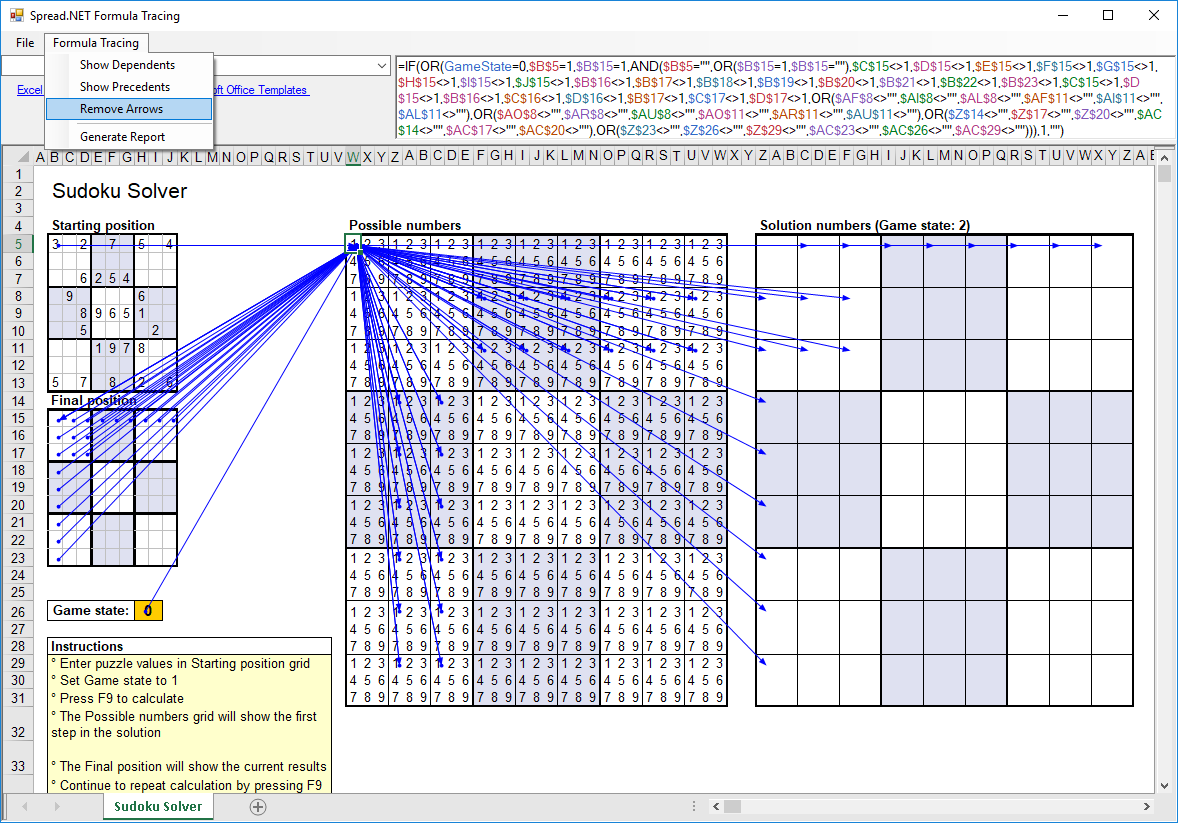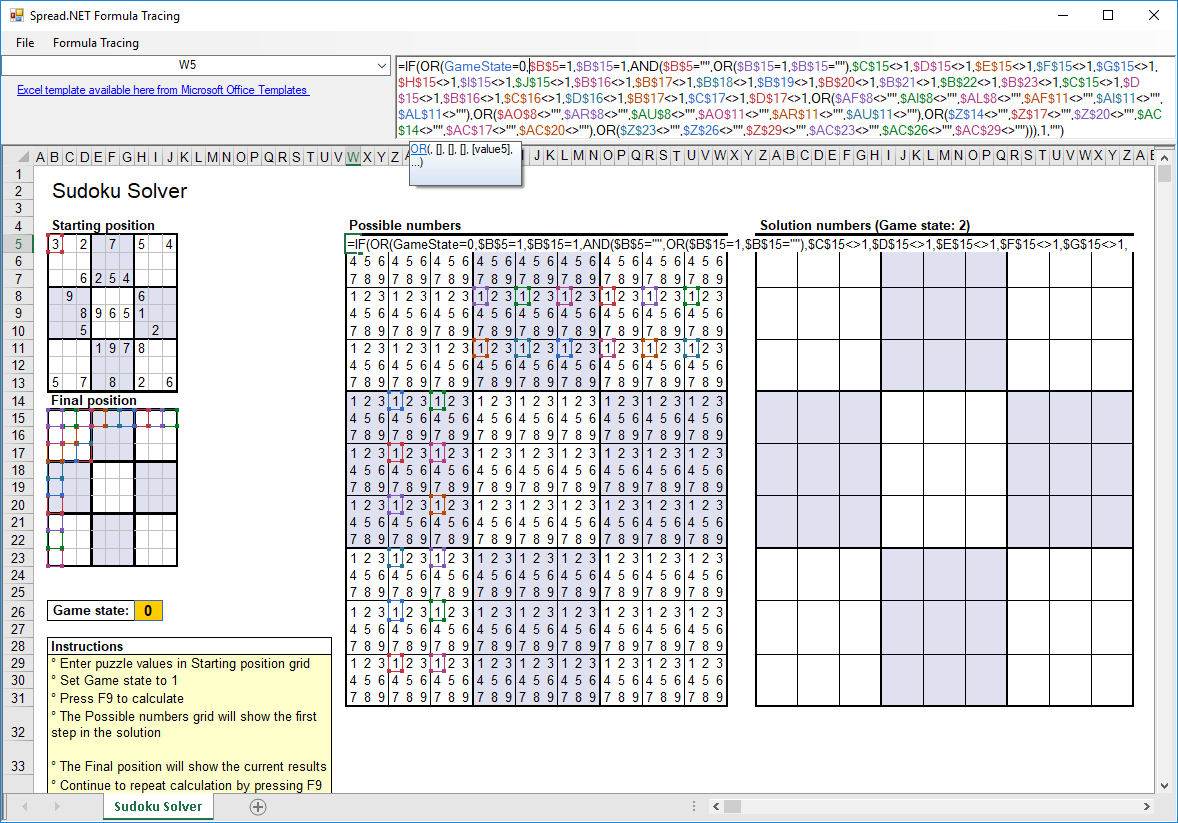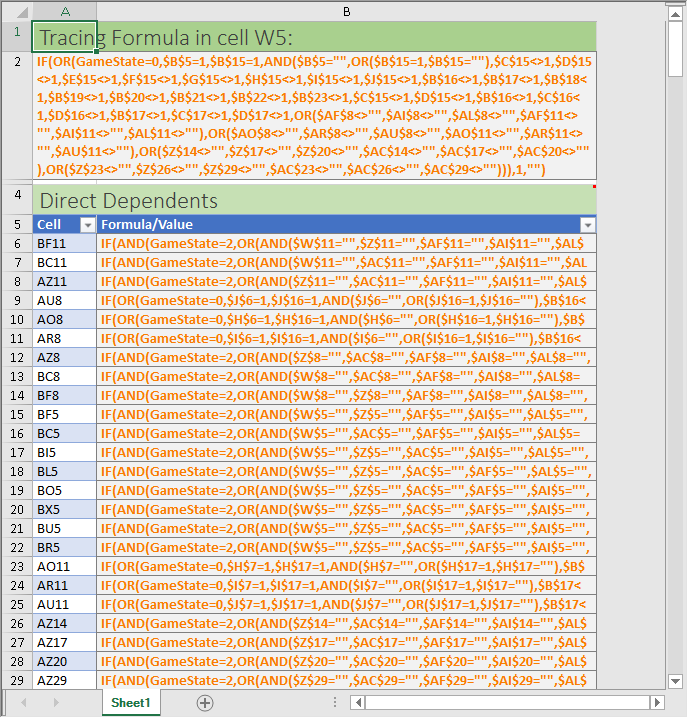﻿ Spread.NET 12 Windows Forms Calculation Part 4 - Formula Tracing and Auditing | Spread# Spread.NET 12 Windows Forms Calculation Part 4 - Formula Tracing and Auditing

Spread.NET 12 Windows Forms introduces a new API layer based on Microsoft Excel's VSTO object model which can be used to implement advanced formula tracing and auditing for spreadsheets. These powerful APIs can return detailed information about the formulas in the worksheets.

Spread.NET 12 Windows Forms has APIs that can:

• Get the formula for a specified cell in a worksheet:
• Get the direct dependent cells for a specified cell, which is the set of cells that contain formulas that reference the specified cell:
• Get the direct precedent cells for a specified cell, which is the set of cells that are referenced by the formula in the specified cell:
• Get all dependent cells for a specified cell, which is the set of cells that contain formulas that reference the specified cell, and all cells which contain formulas that reference any of those cells, and so on until all dependent cells of the specified cell are included:
• Get all precedent cells for a specified cell, which is the set of cells that are referenced by the formula in the specified cell, and also all cells which are referenced by the formulas in those cells, and so on until all precedent cells of the specified cell are included:

The IRange returned by DirectDependents, DirectPrecedents, Dependents, and Precedents may be a multiple selection (a union of disjoint range objects) if there is more than one range to return.

Spread.NET also has built-in tools for tracing formula dependents and precedents using arrow indicators, like Microsoft Excel. To use these tools in your application, you must use the FormulaTextBox control attached to the FpSpread control using the Attach method. Using these APIs, your application can:

• Show arrows from the specified cell to all dependent cells in the current worksheet:
• Show arrows from the specified cell to all precedent cells in the current worksheet:

These auditing tools can be very useful in many important cases:

• Auditing and validating the cell formulas of a very important workbook, for example, business processes or public company financials worksheets used for reporting
• Validating complex logical models
• Finding errors or omissions in calculations
• Figuring out how the complex formulas of an unfamiliar workbook operateThe Spread.NET Formula Tracing sample demonstrates the power of the auditing tools available for tracing the dependencies and precedents of cell formulas. To illustrate the power of these APIs, I selected a template spreadsheet for solving Sudoku puzzles that is available freely on the Microsoft Office templates site here. The image in Figure 1 shows the dependencies and precedents for the cell W5. The formula in this cell is long and complex, and contains many cell references:

``````=IF(OR(GameState=0,\$B\$5=1,\$B\$15=1,AND(\$B\$5="",OR(\$B\$15=1,\$B\$15=""),\$C\$15<>1,\$D\$15<>1,\$E\$15<>1,\$F\$15<>1,\$G\$15<>1,\$H\$15<>1,\$I\$15<>1,\$J\$15<>1,\$B\$16<>1,\$B\$17<>1,\$B\$18<>1,\$B\$19<>1,\$B\$20<>1,\$B\$21<>1,\$B\$22<>1,\$B\$23<>1,\$C\$15<>1,\$D\$15<>1,\$B\$16<>1,\$C\$16<>1,\$D\$16<>1,\$B\$17<>1,\$C\$17<>1,\$D\$17<>1,OR(\$AF\$8<>"",\$AI\$8<>"",\$AL\$8<>"",\$AF\$11<>"",\$AI\$11<>"",\$AL\$11<>""),OR(\$AO\$8<>"",\$AR\$8<>"",\$AU\$8<>"",\$AO\$11<>"",\$AR\$11<>"",\$AU\$11<>""),OR(\$Z\$14<>"",\$Z\$17<>"",\$Z\$20<>"",\$AC\$14<>"",\$AC\$17<>"",\$AC\$20<>""),OR(\$Z\$23<>"",\$Z\$26<>"",\$Z\$29<>"",\$AC\$23<>"",\$AC\$26<>"",\$AC\$29<>""))),1,"")
``````

However, a quick glance at the indicator arrows shows that this formula's precedents – the cells which are referenced by the formula – include the "Game state" cell (H26), the top-left cell in the “Starting position” block (B5), and three sets of cells in the "Final position" block – the cells in the first row (B15:J15), the cells in the first column (B15:B23), and the cells in the first 3x3 "big box" (B15:D17).

It is also clear that this formula's dependents – the cells which reference the formula's calculated value – include certain particular cells in the "1" position inside the "Possible numbers" block, and certain particular cells in the "1" position inside the "Solution numbers" block. The pattern of which of those dependent cells are referenced shows how the logic of the solver operates using the adjacent "1" position cells in the "Possible numbers" block to set the "1" position numbers in the "Solution numbers" block.

You can explore the other formulas in the solver to see how it operates in detail. The cells in the "Final Position" and "Solution numbers" blocks are similarly complex and the indicators are very helpful.

Editing the FormulaTextBox is another powerful tool for viewing the precedents, as the references are color-coded and indicated in the worksheet with corresponding colored cell borders around the referenced range:You can generate a detailed report of the selected cell formula listing each direct dependency, direct precedent, and if there are more dependencies or precedents, the full listing of all dependents and precedents of the formula using the menu item Formula Tracing – Generate Report: (please note, you must first select a cell containing a formula).The generated report shows the cell and formula being traced, and then lists all direct dependents and direct precedents. If there are more dependents than already listed in the direct dependents, then all dependents are listed, and if there are more precedents than the direct precedents then all precedents are listed.

You can trace the formula references in other spreadsheets using the File – Open menu item to open the Excel spreadsheet document (XLSX, XLSM, or XLS).

## The Sample Code

The sample uses the typical layout of docked splitters, as shown in the QuickStart Tutorial in the Spread.NET Control Explorer, with an additional splitter for the main window to show the Formula Tracing Report. The template SudokuSolver.XLSX is loaded from a resource file that is built into the EXE using code in the form constructor, and then loaded with a simple sudoku puzzle that this solver can calculate:

``````[VB]
Public Sub New()
InitializeComponent()
Using s As System.IO.MemoryStream = New
System.IO.MemoryStream(Resources.SudokuSolver)
' fix up columns
Dim wb As IWorkbook = fpSpread1.AsWorkbook()
Dim ws As IWorksheet = wb.ActiveSheet
' intialize a puzzle
Const O As Object = Nothing
ws.SetValue(4, 1, New Object(,) { {3, O, 2, O, 7, O, 5, O, 4},
{O, O, O, O, O, O, O, O, O},
{O, O, 6, 2, 5, 4, O, O, O},
{O, 9, O, O, O, O, 6, O, O},
{O, O, 8, 9, 6, 5, 1, O, O},
{O, O, 5, O, O, O, O, 2, O},
{O, O, O, 1, 9, 7, 8, O, O},
{O, O, O, O, O, O, O, O, O},
{5, O, 7, O, 8, O, 2, O, 6} })
ws.Columns(1).AutoFit()
ws.Columns(22).AutoFit()
ws.Columns(30).AutoFit()
ws.Columns(39).AutoFit()
ws.Columns(48).AutoFit()
ws.Columns(52).AutoFit()
' unprotect sheet so formulas can be edited
ws.Unprotect(Nothing)
' setup iterations And calculation hokey
wb.WorkbookSet.CalculationEngine.Iterative = True
wb.WorkbookSet.CalculationEngine.MaximumIterations = 1
Keystroke(Keys.F9, Keys.None), "Recalculate")
End Using
End Sub
``````
`````` [C#]
public Form1()
{
InitializeComponent();
using (System.IO.MemoryStream s = new
System.IO.MemoryStream(Resources.SudokuSolver))
{
// fix up columns
IWorksheet ws = wb.ActiveSheet;
// intialize a puzzle
const object O = null;
ws.SetValue(4, 1, new object[,] { { 3, O, 2, O, 7, O, 5, O, 4 },
{ O, O, O, O, O, O, O, O, O },
{ O, O, 6, 2, 5, 4, O, O, O },
{ O, 9, O, O, O, O, 6, O, O },
{ O, O, 8, 9, 6, 5, 1, O, O },
{ O, O, 5, O, O, O, O, 2, O },
{ O, O, O, 1, 9, 7, 8, O, O },
{ O, O, O, O, O, O, O, O, O },
{ 5, O, 7, O, 8, O, 2, O, 6 } });
ws.Columns.AutoFit();
ws.Columns.AutoFit();
ws.Columns.AutoFit();
ws.Columns.AutoFit();
ws.Columns.AutoFit();
ws.Columns.AutoFit();
// unprotect sheet so formulas can be edited
ws.Unprotect(null);
// setup iterations and calculation hokey
wb.WorkbookSet.CalculationEngine.Iterative = true;
wb.WorkbookSet.CalculationEngine.MaximumIterations = 1;
Keystroke(Keys.F9, Keys.None), "Recalculate");
}
}
``````

To make the F9 key recalculate the worksheet, a simple custom action class implements the Recalculate action using SheetView.Recalculate:

``````[VB]
Public Class RecalculateAction
Public Overrides Sub PerformAction(sender As Object)
sv.Sheets(sv.ActiveSheetIndex).Recalculate()
End Sub
End Class
``````
``````[C#]
{
public override void PerformAction(object sender)
{
sv.Sheets[sv.ActiveSheetIndex].Recalculate();
}
}
``````

The code to handle the File – Open menu item uses the OpenExcel method and OpenFileDialog, with some extra logic to unprotect the sheets:

``````[VB]
Private Sub OpenToolStripMenuItem_Click(ByVal sender As Object, ByVal e As
EventArgs)
Dim ofd As OpenFileDialog = New OpenFileDialog()
Files (*.*)|*.*"
ofd.FilterIndex = 0

If DialogResult.OK = ofd.ShowDialog() Then
' unprotect sheets so formulas can be edited
For Each sv As SheetView In fpSpread1.Sheets
sv.Protect = False
Next sv
End If
End Sub
``````
``````[C#]
private void OpenToolStripMenuItem_Click(object sender, EventArgs e)
{
OpenFileDialog ofd = new OpenFileDialog();
Files (*.*)|*.*";
ofd.FilterIndex = 0;
if (DialogResult.OK == ofd.ShowDialog())
{
// unprotect sheets so formulas can be edited
foreach ( SheetView sv in fpSpread1.Sheets)
sv.Protect = false;
}
}
``````

The code to handle the menu items Formula Tracing – Show Dependents, Formula Tracing – Show Precedents, and Formula Tracing – Remove Arrows are implemented using the APIs for ShowDependents, ShowPrecedents, HideDependents, and HidePrecedents:

``````[VB]
Private Sub ShowDependentsToolStripMenuItem_Click(ByVal sender As Object, ByVal e
As EventArgs)
End Sub
Private Sub ShowPrecedentsToolStripMenuItem_Click(ByVal sender As Object, ByVal e
As EventArgs)
End Sub
Private Sub RemoveArrowsToolStripMenuItem_Click(ByVal sender As Object, ByVal e
As EventArgs)
For i As Integer = 0 To fpSpread1.Sheets.Count - 1
Next
End Sub
``````
``````[C#]
private void ShowDependentsToolStripMenuItem_Click(object sender, EventArgs e)
{
}
private void ShowPrecedentsToolStripMenuItem_Click(object sender, EventArgs e)
{
}
private void RemoveArrowsToolStripMenuItem_Click(object sender, EventArgs e)
{
for (int i = 0; i < fpSpread1.Sheets.Count; i++)
{
}
}
``````

The code for Formula Tracing – Generate Report uses the APIs for IRange to query the dependent and precedent cells and generate the report. Some IRange objects returned may be multirange selections that must be split using the API for IAreas. The code also takes into account whether the target cell contains a regular formula or an array formula, and uses the appropriate API:

``````[VB]
Private Sub GenerateReportToolStripMenuItem_Click(ByVal sender As Object, ByVal e
As EventArgs)
Dim row, rowStart As Integer
Dim wb As IWorkbook = fpSpread1.AsWorkbook()
Dim wb2 As IWorkbook = fpSpread2.AsWorkbook()
Dim wks As IWorksheet = wb2.ActiveSheet
Dim targetCell As IRange =
If Not CBool(targetCell.HasFormula) Then
MessageBox.Show("You must select a cell containing a formula to analyze
first!")
Return
End If
Dim direct_dependents As IAreas = targetCell.DirectDependents.Areas
Dim direct_precedents As IAreas = targetCell.DirectPrecedents.Areas
Dim all_dependents As IAreas = targetCell.Dependents.Areas
Dim all_precedents As IAreas = targetCell.Precedents.Areas
Dim sv As SheetView = fpSpread2.ActiveSheet
sv.RowCount = Integer.MaxValue
sv.Cells(0, 0).Text = "Tracing Formula in cell " & targetCell.Address(False,
False, ReferenceStyle.A1, False, targetCell) & ":"
wks.Cells(0, 0).ApplyStyle(BuiltInStyle.Percent60Accent6)
wks.Cells(0, 0).ApplyStyle(BuiltInStyle.Title)
wks.Rows(0).AutoFit()
sv.Cells(1, 0).Text = If(targetCell.HasArray, targetCell.FormulaArray,
targetCell.Formula)
sv.Cells(1, 0).ColumnSpan = 2
wks.Cells(1, 0).Style.VerticalAlignment = VerticalAlignment.Top
wks.Cells(1, 0).Style.WrapText = True
sv.SetRowHeight(1, sv.GetPreferredCellSize(1, 0).Height)
wks.Cells(1, 0).ApplyStyle(BuiltInStyle.Percent20Accent6)
wks.Cells(1, 0).ApplyStyle(BuiltInStyle.Calculation)
row = 3
sv.Cells(row, 0).Text = "Direct Dependents"
sv.Cells(row, 0).Note = "Direct Depedents are cells which are directly
referenced in the target cell formula."
sv.Cells(row, 0).ColumnSpan = 2
wks.Cells(row, 0).ApplyStyle(BuiltInStyle.Percent40Accent6)
wks.Cells(row, 0).ApplyStyle(BuiltInStyle.Title)
wks.Rows(row).RowHeight = wks.Rows(0).RowHeight
row += 1
rowStart = row
sv.Cells(row, 0).Text = "Cell"
sv.Cells(row, 1).Text = "Formula/Value"
row += 1
Dim i As Integer = 0
While i < direct_dependents.Count
ReferenceStyle.A1, False, direct_dependents(i))
sv.Cells(row, 1).Text = If(CBool(direct_dependents(i).HasFormula),
(If(direct_dependents(i).HasArray, direct_dependents(i).FormulaArray,
direct_dependents(i).Formula)), direct_dependents(i).Text)
row += 1
i += 1
End While
sv.AddTable(rowStart, 0, row - rowStart, 2)
For i = 0 To direct_dependents.Count - 1
If CBool(direct_dependents(i).HasFormula) Then
wks.Cells(rowStart + 1 + i, 1).ApplyStyle(BuiltInStyle.Calculation)
wks.Cells(rowStart + 1 + i, 1).Style.WrapText = True
sv.SetRowHeight(rowStart + 1 + i, sv.GetPreferredCellSize(rowStart +
1 + i, 1).Height)
End If
Next
row += 2
sv.Cells(row, 0).Text = "Direct Precedents"
sv.Cells(row, 0).Note = "Direct Precedents are cells which directly reference
the target cell."
sv.Cells(row, 0).ColumnSpan = 2
wks.Cells(row, 0).ApplyStyle(BuiltInStyle.Percent40Accent6)
wks.Cells(row, 0).ApplyStyle(BuiltInStyle.Title)
wks.Rows(row).RowHeight = wks.Rows(0).RowHeight
row += 1
rowStart = row
sv.Cells(row, 0).Text = "Cell"
sv.Cells(row, 1).Text = "Formula/Value"
row += 1
i = 0
While i < direct_precedents.Count
ReferenceStyle.A1, False, direct_precedents(i))
sv.Cells(row, 1).Text = If(CBool(direct_precedents(i).HasFormula),
(If(direct_precedents(i).HasArray, direct_precedents(i).FormulaArray,
direct_precedents(i).Formula)), direct_precedents(i).Text)
row += 1
i += 1
End While
sv.AddTable(rowStart, 0, row - rowStart, 2)
For i = 0 To direct_precedents.Count - 1
If CBool(direct_precedents(i).HasFormula) Then
wks.Cells(rowStart + 1 + i, 1).ApplyStyle(BuiltInStyle.Calculation)
wks.Cells(rowStart + 1 + i, 1).Style.WrapText = True
sv.SetRowHeight(rowStart + 1 + i, sv.GetPreferredCellSize(rowStart +
1 + i, 1).Height)
End If
Next
If all_dependents.Count > direct_dependents.Count Then
row += 2
sv.Cells(row, 0).Text = "All Dependents"
sv.Cells(row, 0).Note = "Depedents are cells which are directly
referenced in the target cell formula, and all cells which are directly referenced in
those cell formulas, and so on."
sv.Cells(row, 0).ColumnSpan = 2
wks.Cells(row, 0).ApplyStyle(BuiltInStyle.Percent40Accent6)
wks.Cells(row, 0).ApplyStyle(BuiltInStyle.Title)
wks.Rows(row).RowHeight = wks.Rows(0).RowHeight
row += 1
rowStart = row
sv.Cells(row, 0).Text = "Cell"
sv.Cells(row, 1).Text = "Formula/Value"
row += 1
i = 0
While i < all_dependents.Count
ReferenceStyle.A1, False, all_dependents(i))
sv.Cells(row, 1).Text = If(CBool(all_dependents(i).HasFormula),
(If(all_dependents(i).HasArray, all_dependents(i).FormulaArray,
all_dependents(i).Formula)), all_dependents(i).Text)
row += 1
i += 1
End While
sv.AddTable(rowStart, 0, row - rowStart, 2)

For i = 0 To all_dependents.Count - 1
If CBool(all_dependents(i).HasFormula) Then
wks.Cells(rowStart + 1 + i,
1).ApplyStyle(BuiltInStyle.Calculation)
wks.Cells(rowStart + 1 + i, 1).Style.WrapText = True
sv.SetRowHeight(rowStart + 1 + i, sv.GetPreferredCellSize(rowStart + 1 + i, 1).Height)
End If
Next
End If
If all_precedents.Count > direct_precedents.Count Then
row += 2
sv.Cells(row, 0).Text = "All Precedents"
sv.Cells(row, 0).Note = "Precedents are cells which directly reference
the target cell, and all cells which directly reference those cells, and so on."
sv.Cells(row, 0).ColumnSpan = 2
wks.Cells(row, 0).ApplyStyle(BuiltInStyle.Percent40Accent6)
wks.Cells(row, 0).ApplyStyle(BuiltInStyle.Title)
wks.Rows(row).RowHeight = wks.Rows(0).RowHeight
row += 1
rowStart = row
sv.Cells(row, 0).Text = "Cell"
sv.Cells(row, 1).Text = "Formula/Value"
row += 1
i = 0
While i < all_precedents.Count
ReferenceStyle.A1, False, all_precedents(i))
sv.Cells(row, 1).Text = If(CBool(all_precedents(i).HasFormula),
(If(all_precedents(i).HasArray, all_precedents(i).FormulaArray,
all_precedents(i).Formula)), all_precedents(i).Text)
row += 1
i += 1
End While
sv.AddTable(rowStart, 0, row - rowStart, 2)

For i = 0 To all_precedents.Count - 1
If CBool(all_precedents(i).HasFormula) Then
wks.Cells(rowStart + 1 + i,
1).ApplyStyle(BuiltInStyle.Calculation)
wks.Cells(rowStart + 1 + i, 1).Style.WrapText = True
sv.SetRowHeight(rowStart + 1 + i,
sv.GetPreferredCellSize(rowStart + 1 + i, 1).Height)
End If
Next
End If
wks.RowCount = sv.GetLastNonEmptyRow(NonEmptyItemFlag.Data) + 1
sv.SetColumnWidth(1, 500)
sv.ColumnCount = 2
splitContainer3.Panel2Collapsed = False
End Sub
``````
``````[C#]
private void GenerateReportToolStripMenuItem_Click(object sender, EventArgs e)
{
int row, rowStart;
IWorksheet wks = wb2.ActiveSheet;
IRange targetCell =
if (!(bool)targetCell.HasFormula)
{
MessageBox.Show("You must select a cell containing a formula to analyze
first!");
return;
}
IAreas direct_dependents = targetCell.DirectDependents.Areas;
IAreas direct_precedents = targetCell.DirectPrecedents.Areas;
IAreas all_dependents = targetCell.Dependents.Areas;
IAreas all_precedents = targetCell.Precedents.Areas;
sv.Reset();
sv.RowCount = int.MaxValue;
sv.Cells[0, 0].Text = "Tracing Formula in cell " + targetCell.Address(false,
false, ReferenceStyle.A1, false, targetCell) + ":";
wks.Cells[0, 0].ApplyStyle(BuiltInStyle.Percent60Accent6);
wks.Cells[0, 0].ApplyStyle(BuiltInStyle.Title);
wks.Rows.AutoFit();
sv.Cells[1, 0].Text = targetCell.HasArray ? targetCell.FormulaArray :
targetCell.Formula;
sv.Cells[1, 0].ColumnSpan = 2;
wks.Cells[1, 0].Style.VerticalAlignment = VerticalAlignment.Top;
wks.Cells[1, 0].Style.WrapText = true;
sv.SetRowHeight(1, sv.GetPreferredCellSize(1, 0).Height);
wks.Cells[1, 0].ApplyStyle(BuiltInStyle.Percent20Accent6);
wks.Cells[1, 0].ApplyStyle(BuiltInStyle.Calculation);

row = 3;
sv.Cells[row, 0].Text = "Direct Dependents";
sv.Cells[row, 0].Note = "Direct Depedents are cells which directly reference
the target cell.";
sv.Cells[row, 0].ColumnSpan = 2;
wks.Cells[row, 0].ApplyStyle(BuiltInStyle.Percent40Accent6);
wks.Cells[row, 0].ApplyStyle(BuiltInStyle.Title);
wks.Rows[row].RowHeight = wks.Rows.RowHeight;
row++;
rowStart = row;
sv.Cells[row, 0].Text = "Cell";
sv.Cells[row, 1].Text = "Formula/Value";
row++;
for (int i = 0; i < direct_dependents.Count; row++, i++ )
{
ReferenceStyle.A1, false, direct_dependents[i]);
sv.Cells[row, 1].Text = (bool)direct_dependents[i].HasFormula ?
(direct_dependents[i].HasArray ? direct_dependents[i].FormulaArray :
direct_dependents[i].Formula) : direct_dependents[i].Text;
}
sv.AddTable(rowStart, 0, row - rowStart, 2);
for (int i = 0; i < direct_dependents.Count; i++)
{
if ((bool)direct_dependents[i].HasFormula)
{
wks.Cells[rowStart + 1 + i, 1].ApplyStyle(BuiltInStyle.Calculation);
wks.Cells[rowStart + 1 + i, 1].Style.WrapText = true;
sv.SetRowHeight(rowStart + 1 + i, sv.GetPreferredCellSize(rowStart +
1 + i, 1).Height);
}
}
row += 2;
sv.Cells[row, 0].Text = "Direct Precedents";
sv.Cells[row, 0].Note = "Direct Precedents are cells which are directly
referenced in the target cell formula.";
sv.Cells[row, 0].ColumnSpan = 2;
wks.Cells[row, 0].ApplyStyle(BuiltInStyle.Percent40Accent6);
wks.Cells[row, 0].ApplyStyle(BuiltInStyle.Title);
wks.Rows[row].RowHeight = wks.Rows.RowHeight;
row++;
rowStart = row;
sv.Cells[row, 0].Text = "Cell";
sv.Cells[row, 1].Text = "Formula/Value";
row++;
for (int i = 0; i < direct_precedents.Count; row++, i++)
{
ReferenceStyle.A1, false, direct_precedents[i]);
sv.Cells[row, 1].Text = (bool)direct_precedents[i].HasFormula ?
(direct_precedents[i].HasArray ? direct_precedents[i].FormulaArray :
direct_precedents[i].Formula) : direct_precedents[i].Text;
}
sv.AddTable(rowStart, 0, row - rowStart, 2);
for (int i = 0; i < direct_precedents.Count; i++)
{
if ((bool)direct_precedents[i].HasFormula)
{
wks.Cells[rowStart + 1 + i, 1].ApplyStyle(BuiltInStyle.Calculation);
wks.Cells[rowStart + 1 + i, 1].Style.WrapText = true;
sv.SetRowHeight(rowStart + 1 + i, sv.GetPreferredCellSize(rowStart +
1 + i, 1).Height);
}
}
if (all_dependents.Count > direct_dependents.Count)
{
row += 2;
sv.Cells[row, 0].Text = "All Dependents";
sv.Cells[row, 0].Note = "Depedents are cells which directly reference the
target cell, and all cells which directly reference those cells, and so on.";
sv.Cells[row, 0].ColumnSpan = 2;
wks.Cells[row, 0].ApplyStyle(BuiltInStyle.Percent40Accent6);
wks.Cells[row, 0].ApplyStyle(BuiltInStyle.Title);
wks.Rows[row].RowHeight = wks.Rows.RowHeight;
row++;
rowStart = row;
sv.Cells[row, 0].Text = "Cell";
sv.Cells[row, 1].Text = "Formula/Value";
row++;
for (int i = 0; i < all_dependents.Count; row++, i++)
{
ReferenceStyle.A1, false, all_dependents[i]);
sv.Cells[row, 1].Text = (bool)all_dependents[i].HasFormula ?
(all_dependents[i].HasArray ? all_dependents[i].FormulaArray :
all_dependents[i].Formula) : all_dependents[i].Text;
}
sv.AddTable(rowStart, 0, row - rowStart, 2);
for (int i = 0; i < all_dependents.Count; i++)
{
if ((bool)all_dependents[i].HasFormula)
{
wks.Cells[rowStart + 1 + i,
1].ApplyStyle(BuiltInStyle.Calculation);
wks.Cells[rowStart + 1 + i, 1].Style.WrapText = true;
sv.SetRowHeight(rowStart + 1 + i,
sv.GetPreferredCellSize(rowStart + 1 + i, 1).Height);
}
}
}
if (all_precedents.Count > direct_precedents.Count)
{
row += 2;
sv.Cells[row, 0].Text = "All Precedents";
sv.Cells[row, 0].Note = "Precedents are cells which are directly
referenced in the target cell formula, and all cells which are directly referenced in
those cell formulas, and so on.";
sv.Cells[row, 0].ColumnSpan = 2;
wks.Cells[row, 0].ApplyStyle(BuiltInStyle.Percent40Accent6);
wks.Cells[row, 0].ApplyStyle(BuiltInStyle.Title);
wks.Rows[row].RowHeight = wks.Rows.RowHeight;
row++;
rowStart = row;
sv.Cells[row, 0].Text = "Cell";
sv.Cells[row, 1].Text = "Formula/Value";
row++;
for (int i = 0; i < all_precedents.Count; row++, i++)
{
ReferenceStyle.A1, false, all_precedents[i]);
sv.Cells[row, 1].Text = (bool)all_precedents[i].HasFormula ?
(all_precedents[i].HasArray ? all_precedents[i].FormulaArray :
all_precedents[i].Formula) : all_precedents[i].Text;
}
sv.AddTable(rowStart, 0, row - rowStart, 2);
for (int i = 0; i < all_precedents.Count; i++)
{
if ((bool)all_precedents[i].HasFormula)
{
wks.Cells[rowStart + 1 + i,
1].ApplyStyle(BuiltInStyle.Calculation);
wks.Cells[rowStart + 1 + i, 1].Style.WrapText = true;
sv.SetRowHeight(rowStart + 1 + i,
sv.GetPreferredCellSize(rowStart + 1 + i, 1).Height);
}
}
}
wks.RowCount = sv.GetLastNonEmptyRow(NonEmptyItemFlag.Data) + 1;
sv.SetColumnWidth(1, 500);
sv.ColumnCount = 2;
splitContainer3.Panel2Collapsed = false;
}
``````

#### VB sample code | C# sample code

In another article, we show you how to implement a custom function for Spread.NET 12 Windows Forms (that works just like the built-in functions in cell formulas.

Thanks for following along, happy coding!